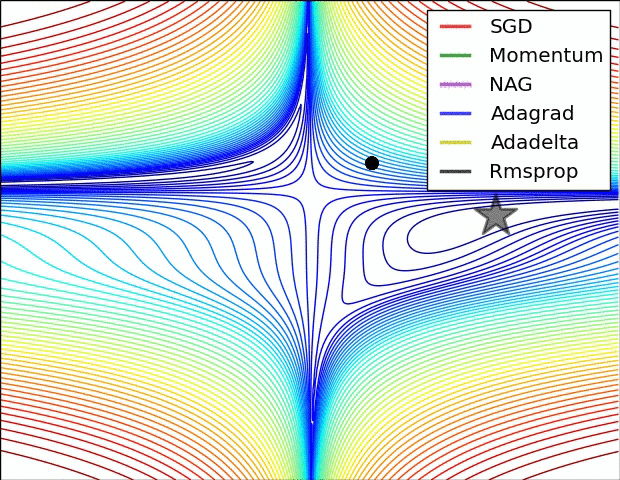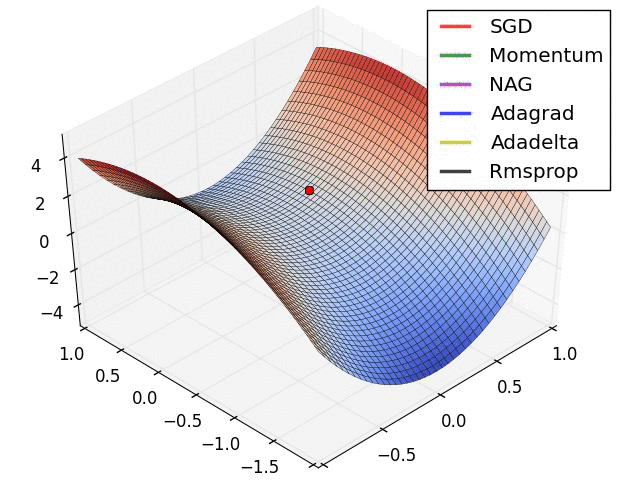# 梯度下降优化算法

• 梯度下降法
• 梯度下降优化
o 均方根支(Root Mean Square Prop, RMSprop)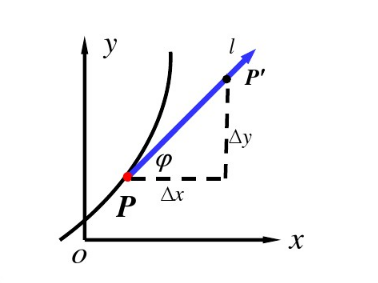(1)∂f∂l=limρ→0f(x+Δx,y+Δy)−f(x,y)ρ

f′(x0)=limx→x0f(x)−f(x0)x−x0=limΔx→0f(x0+Δx)−f(x0)Δx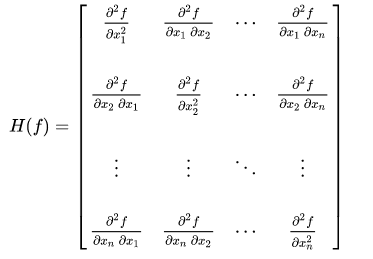H(x)=[∂2f∂xi∂xj]n×n

• 正定矩阵：所有特征值大于0 (>0)
• 负定矩阵：所有特征值小于0 (<0)
• 半正定矩阵： 所有特征值为非负（>=0）
• 半负定矩阵：所有特征值为非正（<=0）
• 不定矩阵：特征值有正有负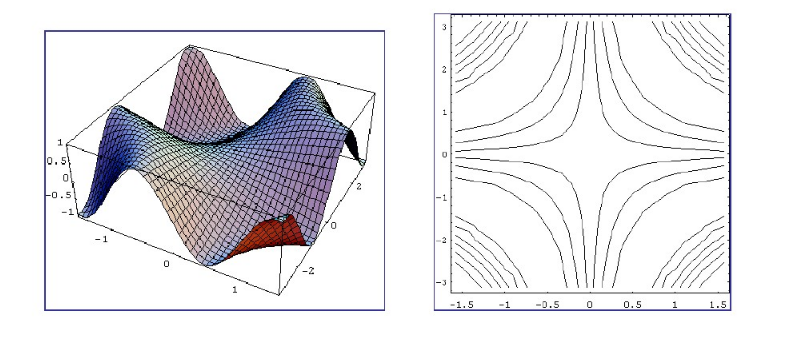J(w,b)=1m∑i=1mL(y(i)^,y(i))+λ2m∑∥w∥F2wj:=wj−α∂J(w,b)∂wjbj:=bj−α∂J(w,b)∂bj

BGD的梯度下降图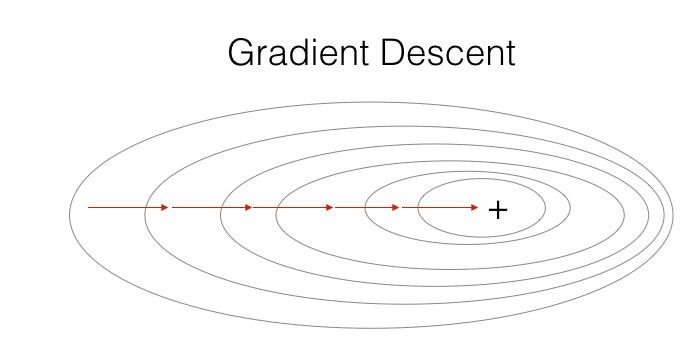BGD的优缺点
• 优点：最小化所有训练样本的损失函数得到全局最优
• 缺点：当样本数目很多时，训练过程很慢
Python伪代码
1
2
3
4 for epoch in range(epochs):
# 是对每个epoch的所有数据进行计算的
params = params - learning_rate * grad

J(w,b)=L(y(i)^,y(i))+λ2∑∥w∥F2wj:=wj−α∂J(w,b)∂wjbj:=bj−α∂J(w,b)∂bj
SGD的梯度下降图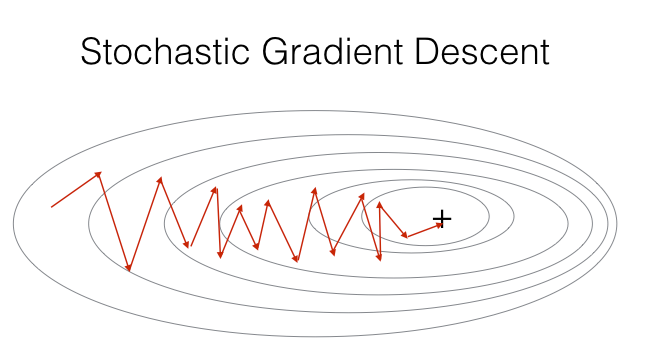SGD的优缺点
• 优点：训练速度快
• 缺点：不易找到全局最优
Python伪代码
1
2
3
4
5 for epoch in range(epochs):
shuffle(data)
for example in data:
params = params - learning_rate * grad

J(w,b)=1k∑i=1kL(y(i)^,y(i))+λ2k∑∥w∥F2wj:=wj−α∂J(w,b)∂wjbj:=bj−α∂J(w,b)∂bj
MBGD的梯度下降图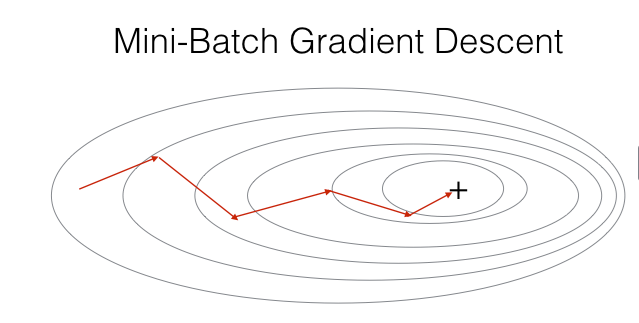Python伪代码
1
2
3
4
5 for epoch in range(epochs):
shuffle(data)
for batch in next_batch(data, batch_size):
params = params - learning_rate * grad

• 算法
• 优化方法的选择，比如大数据量下采用牛顿法/拟牛顿法进行优化

St={Y1 if t=1βSt−1+(1−β)Yt if t>1
Yt 为 t下的实际值， St 为 t 下加权平均后的值， β 为权重

υdw=βυdw+(1−β)dwυdb=βυdb+(1−β)dbw:=w−αυdwb:=b−αυdb
SGD 在局部沟壑中很容易发生振荡，所以在这种情况下下降速度会很慢，而动量能在一定程度上抑制这种震荡，使得SGD的下降更平稳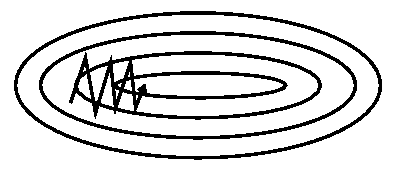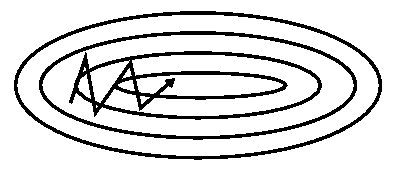Sdw=βSdw+(1−β)dw2Sdb=βSdb+(1−β)db2w:=w−αdwSdw+ϵb:=b−αdwSdb+ϵ
ϵ 一般值很小，主要是用来提高数值稳定性，防止分母过小

υdw=β1υdw+(1−β1)dw,υdb=β1υdb+(1−β1)dbSdw=β2Sdw+(1−β2)dw2,Sdb=β2Sdb+(1−β2)db2υdwcorrect=υdw1−β1t,υdbcorrect=υdb1−β1tSdwcorrect=Sdw1−β2t,Sdbcorrect=Sdb1−β2tw:=w−αυdwcorrectSdwcorrect+ϵb:=b−αυdbcorrectSdbcorrect+ϵ
β1为第一阶矩，β2 为第二阶矩